# PSEB 5th Class Maths Solutions Chapter 6 Measurement Ex 6.2

Punjab State Board PSEB 5th Class Maths Book Solutions Chapter 6 Measurement Ex 6.2 Textbook Exercise Questions and Answers.

## PSEB Solutions for Class 5 Maths Chapter 6 Measurement Ex 6.2

1. Find the weight.

Question 1.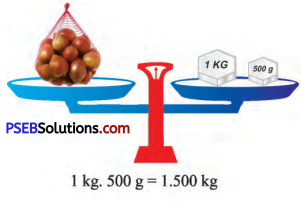Question 2.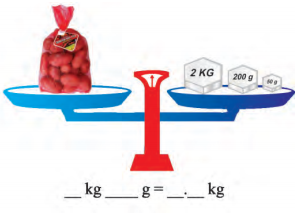Solution:
2 kg 250g = 2.250 kgQuestion 3.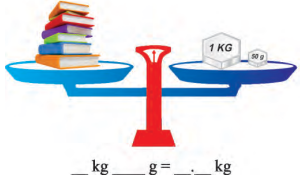Solution:
1 kg 50 g = 1.50 kg

Question 4.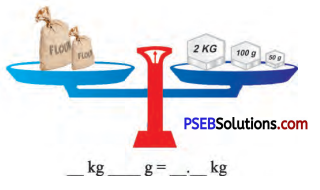Solution:
2 kg 150 g = 2.150 kg

Question 2.
Tick ( ✓) the required weights for the following :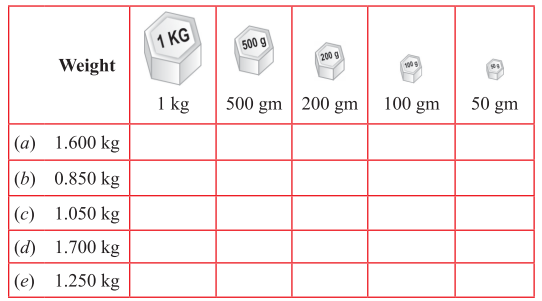Solution: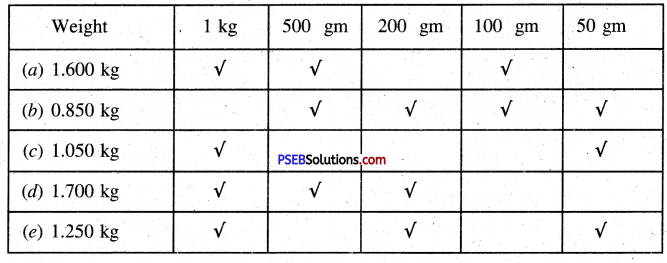3. Fill in the blanks :

Question 1.
2.850 kg = ……………….. kg …………… g
Solution:
2.850 kg = 2 kg 850 g

Question 2.
15.790 g = …………… g ……………….. mg
Solution:
15.790 g = 15 g 790 mgQuestion 3.
……………….. kg = 12 kg 625 g
Solution:
12.625 kg = 12 kg 625 g

Question 4.
……………….. kg = 7 kg 75 g
Solution:
7.075 kg = 7 kg 75 g

Question 5.
……………….. g = 10 g 800 mg
Solution:
10.800 g = 10 g 800 mg

4. Convert :

Question 1.
3.275 g into mg
Solution:
3.275 g = 3.275 × 1000 mg = 3275 mgQuestion 2.
8050 g into kg
Solution:
8050 g = $$\frac{8050}{1000}$$ kg= 8.050 kg

Question 3.
4.2 kg into g
Solution:
4.2 kg = 4.2 × 1000 g = 4200 g

Question 4.
865 mg into g
Solution:
865 mg = $$\frac{865}{1000}$$ g = 0.865 gQuestion 5.
520 g into kg
Solution:
520 g = $$\frac{520}{1000}$$ kg = 0.520 kg